## Introduction

Complex networks are increasingly being investigated in various fields of nature and society1,2,3,4,5 and from different angles, such as collective behaviour6,7,8,9,10, robustness11,12, controllability13,14, etc. Many findings have been revealed through the discovery of statistical and topological properties of complex networks or via modelling and analysing network behaviours (or characteristics). All of these results can help us well explain distinct phenomena of complex networks in some ways. However, more challenges remain to fully understand complex networks. Cascading failure is one of the fascinating and significant challenges.

Large cascades are common in most complex networks15. Earlier works regarding the cascading process in complex networks focused on failures triggered just by the removal of a single node16 or small initial shocks17. Recently, the dependency property, modelled by dependency links18,19,20, has been proposed to study the effects of dependency among nodes on the evolution of complex networks, especially for cascading failures. One of the characteristics discriminating them from the traditional connectivity links is that dependent links present the relations among local nodes, not the global topological relations of a network. According to ref. 18, dependency studies usually focus either on failures due to overloads in networks or on local dependency models in which each node’s state depends only on the states of its neighbours and therefore a failing node will also cause its neighbours to fail, etc. After dependency groups were proposed, many researchers expanded these studies to random dependency models. One fundamental difference between local dependency models and random dependency models is that dependencies between nodes are either confined to adjacent nodes or not.

From previous discussions, static dependency is a common assumption when investigating the dependency property. One key reason is that the dependency relations among nodes are considered to be fixed during the process of cascading failure, which simplifies the theoretical analysis of cascading failures tactfully regarding the dependency property. Yet, it seems more appropriate to introduce dynamical dependency groups to network evolution partially due to the ubiquitousness of the dynamical dependency property in real networks. For instance, acquisitions and mergers have easily taken place in almost every industry30, resulting in dynamic changes of dependency groups in financial networks. In social networks, each individual reacts adaptively to its own changing propensities and capabilities, which leads to dynamical dependency groups31. In epidemiological networks, due to more and more frequent social personnel flow, accidentally coming in contact with others gives rise to time-dependent dependency groups. Therefore, it is necessary to explore cascading failures of networks under dynamical dependency.

The dependency property in networks has two sides. It is well known that the dependency property makes these networks more fragile under failure. However, the dependency property contributes to the recovery of failure nodes in dependency groups under certain conditions. In a dependency group, a fraction of nodes exists whose failure has no effect on the function of the group23. These failed nodes can be recovered, called dependency recovery, by means of the dependency property. For example, for communities in social networks, the process of social influence can promote convergence in behaviour32. Conformity behaviour makes the minority swim with the tide. Recently, network recovery mechanisms, such as targeted recovery33, greedy recovery34 and spontaneous recovery35 after a time period, have been investigated with respect to network robustness. All of these efforts indicate that network robustness is the gaming result between failure and the recovery of nodes, especially for networks with large time scale evolution, e.g., social and economic systems17. Therefore, the failure and recovery of dynamical dependency groups coexist in a variety of complex networks, inspiring us to explore how robust or vulnerable these networks behave.

The aim of this study is to determine the effect of dynamical dependency groups on the percolation of networks with a recovery mechanism. Our main contribution can be summarized as follows: (I) We simultaneously introduce dynamical dependency groups and the recovery mechanism to investigate the robustness of networks. By means of a general evolving network model, we can understand the cascading failures of real networks better, where varying dependency relation is widespread. Then, we find that the dynamical dependency among nodes results in disintegrating the network in the form of an abrupt phase transition after an initial random failure of nodes. (II) We consider the robustness discussed here as the gaming result between the failure mechanisms and recovery mechanisms of a network. Through our analysis, we find that dependency strength has a nonlinear effect on network robustness, which is significantly different from the linear effect on networks with static dependency. This robustness, reflecting the capacity of real networks against failure, provides insight to the network designer. This article is organized as follows. In the section of results, firstly, we present an evolving network model consisting of failure mechanisms, a recovery mechanism and a dynamic mechanism of dependency groups. Then, we investigate the effects of dependency strength and the size of the dependency group on network robustness. The relevant discussions are given in the next section. By employing generating function techniques, we give analytical results based on our presented model in the methods section.

## Results

### An Evolving Network Model with Dynamical Dependency

We here introduce a generic evolving model with dynamical dependency groups under the coexistence of cascading failure and a recovery mechanism as a basis of our theoretical and numerical analysis. Consider an arbitrary network of size N with degree distribution Pk. Initially, a fraction q of nodes is chosen randomly to form dependency groups with size g; all other nodes with the fraction (1 − q) do not belong to any dependency groups. After randomly removing a fraction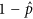of nodes and their links, the failed nodes may cause the other nodes to disconnect from the network (percolation process18). All of the failure nodes are called the initial removal 1 − p. Then, dependency groups undergo the dependency process and dependency evolution.

• Dependency Process. If the fraction of the failure nodes of a group is equal to or larger than the dependency failure threshold, the group will fail, i.e., all nodes in this group will fail, even though they still connect to the network via connectivity links (dependency failure process23). If the fraction of the failure nodes of a group is less than or equal to the dependency recovery threshold, the group will recover, i.e., the failure nodes in this group will go back to normal (dependency recovery process). This dependency process, including the dependency failure process and the dependency recovery process, can be described by the following mathematical model:where pg is the fraction of functional nodes in this group; gF and gR are the failure threshold and the recovery threshold, respectively.

• Dependency Evolution. After one dependency process triggered by failed nodes and its subsequent percolation process, previous dependency groups will evolve into new dependency groups. These new dependency groups still consist of randomly chosen nodes.

Each dependency process and its subsequent percolation process are called one iteration. During one iteration, the failed nodes may trigger more failed nodes, recover partially from failure, or have no effect on other nodes. Therefore, the frequency of group change has no influence on network robustness. If the failed nodes trigger more failed nodes, the frequency only shifts the number of iterations in the cascading process; otherwise, the cascading process stops before the dependency groups change. To facilitate the theoretical analysis, our model assumes that the new dependency groups will replace previous dependency groups between each iteration. Once the cascade process begins, the dependency process and percolation process will occur alternately until there are no further nodes whose state is different from others. Note that under static dependency, our model is equivalent to the failure mechanism proposed by Wang et al.23 when ggR < 1; furthermore, when ggR < 1 and ggF < 1, our model reduces to the representative failure mechanism proposed by Parshani et al.18.

To illustrate our model mentioned above explicitly, we give a typical simple example, as shown in Fig. 1. We find that, by means of simulations, an initial failure of network nodes disintegrates the network in the form of an abrupt transition if the dynamical dependency always exists. The property, i.e., that the cascading process leads to an abrupt transition, exists for a wide range of topologies, including Erdős-Rényi (ER) and Barabási-Albert scale free (BA) networks, indicating that it is a general property of many networks (Fig. 2). This can be explained qualitatively by our model. Those networks with a small fraction of removed nodes will recover from failure. Conversely, due to a large fraction of removed nodes, the entire network will be fragmented.

### Gaming between Failure and Recovery

In reality, how to improve network robustness or protect a network from vulnerability remains an overarching concern. From Fig. 2, we can see that networks with a larger recovery threshold are more robust, while a smaller failure threshold makes the network more vulnerable. It seems good to strengthen robustness by increasing the recovery failure threshold and the failure threshold. However, we cannot continuously increase the recovery threshold and the failure threshold of dependency groups to enhance robustness due to the cost constraint. Under a certain recovery threshold and failure threshold, we pay more attention to identifying the network property with which we can improve network robustness against random failure. Through our analysis in the Methods section, we find that the dependency strength and size of the dependency group play important roles in the cascading process. The evolving behaviour of a network to cascading failure is affected significantly when these parameters are changed. Hence, we investigate the effects of the dependency strength and size of the dependency group on the network robustness.

Regarding the dependency strength of a network, previous studies18,26 have demonstrated that the dependency strength q has a critical effect on the robustness of networks: reducing the dependency strength results in a change from a first-order to a second-order percolation transition. Nevertheless, in our model, the existence of dynamical dependency among nodes can lead to an abrupt percolation phase transition. This is because the synergy between the cascading failure process and the cascading recovery process will result in failure or recovery of the whole network when reaching a steady state. Moreover, it is observed that the dependency strength accelerates the cascading process of networks. Figure 3 shows the fraction of giant component at the end of cascading process α and the number of iterative failures (NOI) of the same networks under different dependency strength. The networks include the ER network (pentagram) and BA network (square). With decreasing dependency strength q, the NOI increases significantly. This can be explained by Eq. (5) in the Methods section; when we reduce the dependency strength q, α will depend less on the dependency part, which will delay the breakdown time. Furthermore, from Eq. (12), we reveal the nonlinear effect of dependency strength on network robustness.

Next, we study how the size of the dependency group affects the network robustness. According to Eq. (12), the critical point pc is dependent on the size g of the dependency group, the recovery threshold gR, the failure threshold gF and the dependency strength q. We all know that the cumulative probability density function of binomial distribution is defined as: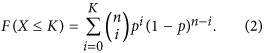It is an increasing function of probability p that increases with the total number of samples n if K > np, while it decreases with n if K < np. Therefore, the critical point pc increases with the size g of the dependency group if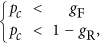and decreases with g ifFurthermore, for cases under the situation pc > gF and pc < 1 − gR, the condition gF < 1 − gR is always met. Thus, we can obtain that pc decreases with g when: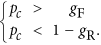In real networks, there are no situations of pc < gF and pc > 1 − gR because, in general, gF > gR.

Roughly speaking, networks with a larger dependency group size are more robust if pc < gF and more vulnerable if pc > gF. Simulation results show the validity of our analysis. In Fig. 4, we plot the values of pc of different size g of an ER network (pentagram) and BA network (square) for a given recovery threshold (0.1) and failure threshold (0.4). In these networks, because pc > gF, pc decreases with size g. This is consistent with the simulation results. Moreover, it has been shown from Fig. 4 that a network with a larger size of dependent groups will more slowly respond to initial removal, which can be reflected by the special behaviour characterizing the NOI in the cascading process. For a larger size of dependent groups, the response approaches steady state much more slowly. This is because the interval of gR < 1 − p < gF increases with the size g of the dependency group, that is, the fraction of the network without dependency groups, which equivalently reduces the dependency strength.

## Discussion

Concerning the dynamical dependency in real networks, we propose a cascading failure model for a network with dynamical dependency groups and a recovery mechanism. In our presented evolving network model, the dependency relation among nodes is time-varying, which is more accordant with real networks, and the failing node could be recovered if the group it belongs to satisfies the recovery condition. Our presented model is more universal because it covers the model in which the failure of one or some nodes can lead to the failure of a dependency group when ggR < 1.

Both simulation and analytical results reveal that the network disintegrates in the form of an abrupt transition. According to analysis, the critical point of our model depends on the size of the dependent group, the dependency strength, the recovery threshold and the failure threshold. Furthermore, we find that, in general, a larger dependency group always makes such a network more vulnerable when the failure threshold gF < pc, whereas for the failure threshold gF > pc, a network with a larger dependency group is more vulnerable. No matter the number of failure and recovery thresholds, a larger group size gives the network more power to resist the initial removal, which indicates that we have more time to recover the failure nodes before the network breaks down. By means of our evolving network model and the analysis, we can have a better understanding of the robustness of a real networked system with the dependency property.

## Methods

To solve this model, the exact analytical solution of the dependency process and the percolation process can be obtained by means of the mean-field approximation and the generating function techniques, respectively. In a mean-field approximation, after initially removing a fraction 1 − p of nodes, 1 − p is the average probability that any node has failed. Let PR and PF be the probability that a dependency group is functioning and failing, respectively. As the setting of our model for the typical case of q = 1, PR and PF can be obtained according to Eq. (1):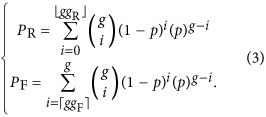where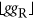is the largest integer smaller than or equal to ggR;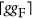is the smallest integer larger than or equal to ggF.

Thus, the fraction of nodes that remain functional after the dependency process, gD(p), is: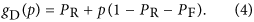Following a similar approach for the general case of 0 ≤ q ≤ 1 yields the universal function gD(p):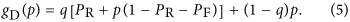Analogous to gD(p), gP(p) is defined as the fraction of nodes belonging to the giant component of the connectivity network after random removal of fraction 1 − p of the nodes. The percolation process can be solved analytically by using the apparatus of generating functions21,36,37. As shown in the generating function methods, we introduce the generating function of the degree distributions G0(ξ) = ∑kP(k)ξk and the generating function of the underlining branching processes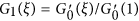. Random removal of 1 − p nodes will change the degree distribution of the remaining nodes; then, the generating function of the new distribution is equal to the generating function of the original distribution, with the argument equal to 1 − p(1 − ξ)36. The fraction of nodes that belongs to the giant component after the removal of 1 − p nodes is ref. 37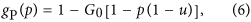where u = u(p) satisfies the self-consistency relation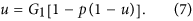It follows from the previous analysis that the network will return back to normal if the number of nodes recovering from failure, ΔTR, is larger than the number of nodes failing, ΔTF, at every iteration; otherwise, if ΔTR < ΔTF, the network will undergo crash. Therefore, it is natural to pay more attention to the critical point pc such that: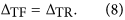Because functions gD(p) and gP(p) are non-decreasing, we can find the critical point of this model at the first iteration. For the first iteration, the number of recovery nodes ΔTR is: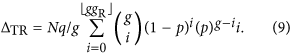The number of failed nodes in the dependency process, ΔTDF, is: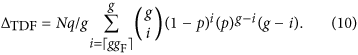After the dependency process, there may be several nodes that need to be removed in the percolation process. Before this percolation process, the accumulated failure, including the initial removal of (1 − p) and the removal of (ΔTR − ΔTF)/N due to the dependency process, is equivalent to a single random removal of [1 − p0 − (ΔTR − ΔTF)/N], where p0 = p/(1 − u), u = G1[1 − p0(1 − u)]. Therefore, the number of failing nodes in the first cascading process, ΔTF, is:According to Eq. (8), the critical point of this model can be obtained. Note that ΔTF or ΔTR is not the real value of every dependency group, just a mean value of all groups. Therefore, phase transition occurs at around the critical point for real networks.

The formalism presented above is generic and applicable to any random network with arbitrary degree distribution. In the case of a common ER random network whose degrees are Poisson distributed38,39, the problem can be solved explicitly. Let the average degree of the network be k. Then, the generating function can be written as G1(ξ) = G0(ξ) = exp [k(ξ − 1)]. Substituting these generating functions into Eqs (6) and (7), we derive gP(p) = 1 − u and u = exp (kp(u − 1)), respectively. Thus, ΔTPF = Np1u, where p1 = p0 + (ΔTR − ΔTF)/N and u = exp (kp1(u − 1)). Furthermore, we get the following equations for the critical point pc: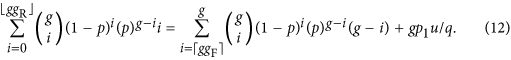Equation (12) gives the relation of the critical point pc, the failure threshold gF, the recovery threshold gR and the dependency strength q. To validate the theoretical results, we carry out simulations on an ER network of size N = 2 × 104 and plot the size of the giant component at the end of the cascading process as a function of the recovery threshold gR in Fig. 5. It is observed from Fig. 5 that the simulation results agree well with the theoretical results.